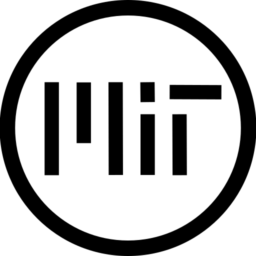# Gaussian Blur Functions for GLES2

Since GLES2 doesn’t have a textureLod() function available in the shaders, i’ve decided to put in the research to port and improve existing functions to replace said method. Here are 4 functions, 2 single-pass, 2 multi-pass, some work better in certain scenarios than others, read the comments inside the code for more insight

``````shader_type canvas_item;

// Xor's gausian blur function
//
// BLUR BLURRINESS (Default 8.0)
// BLUR ITERATIONS (Default 16.0 - More is better but slower)
// BLUR QUALITY (Default 4.0 - More is better but slower)
//
// Desc.: Don't have the best performance but will run on almost
// anything, although, if developing for mobile, is better to use
vec4 texture_xorgaussian(sampler2D tex, vec2 uv, vec2 pixel_size, float blurriness, int iterations, int quality){
float pi = 6.28;

vec2 radius = blurriness / (1.0 / pixel_size).xy;
vec4 blurred_tex = texture(tex, uv);

for(float d = 0.0; d < pi; d += pi / float(iterations)){
for( float i = 1.0 / float(quality); i <= 1.0; i += 1.0 / float(quality) ){
vec2 directions = uv + vec2(cos(d), sin(d)) * radius * i;
blurred_tex += texture(tex, directions);
}
}
blurred_tex /= float(quality) * float(iterations) + 1.0;

return blurred_tex;
}

// Experience-Monks' fast gaussian blur function
//
// BLUR ITERATIONS (Default 16.0 - More is better but slower)
// BLUR DIRECTION (Direction in which the blur is apllied, use vec2(1, 0) for first pass and vec2(0, 1) for second pass)
//
// Desc.: ACTUALLY PRETTY SLOW but still pretty good for custom cinematic
// bloom effects, since this needs render 2 passes
vec4 texture_monksgaussian_multipass(sampler2D tex, vec2 uv, vec2 pixel_size, int iterations, vec2 direction){
vec4 blurred_tex = vec4(0.0);
vec2 resolution = 1.0 / pixel_size;

for (int i=0; i < iterations; i++){
float size = float(iterations - i);

vec2 off1 = vec2(1.3846153846) * (direction * size);
vec2 off2 = vec2(3.2307692308) * (direction * size);

blurred_tex += texture(tex, uv) * 0.2270270270;
blurred_tex += texture(tex, uv + (off1 / resolution)) * 0.3162162162;
blurred_tex += texture(tex, uv - (off1 / resolution)) * 0.3162162162;
blurred_tex += texture(tex, uv + (off2 / resolution)) * 0.0702702703;
blurred_tex += texture(tex, uv - (off2 / resolution)) * 0.0702702703;
}

blurred_tex /= float(iterations) + 1.0;

return blurred_tex;
}

// u/_NoDev_'s gaussian blur function
//
// BLUR BLURRINESS (Default 8.0 - More is better but slower)
// BLUR DIRECTION (Direction in which the blur is apllied, use vec2(1, 0) for first pass and vec2(0, 1) for second pass)
//
// Desc.: Really fast and GOOD FOR MOST CASES, but might NOT RUN IN THE WEB!
// use 'texture_xorgaussian' instead if you found any issues.
vec4 texture_nodevgaussian_singlepass(sampler2D tex, vec2 uv, vec2 pixel_size, float blurriness, float radius){
float pi = 3.14;
float n = 0.0015;

vec4 blurred_tex = vec4(0);

float weight;
for (float i = -blurriness; i <= blurriness; i++){
float d = i / pi;
vec2 anchor = vec2(cos(d), sin(d)) * radius * i;
vec2 directions = uv + pixel_size * anchor;
blurred_tex += texture(tex, directions) * n;
if (i <= 0.0) {n += 0.0015; }
if (i > 0.0) {n -= 0.0015; }
weight += n;
}

float norm = 1.0 / weight;
blurred_tex *= norm;
return blurred_tex;
}
vec4 texture_nodevgaussian_multipass(sampler2D tex, vec2 uv, vec2 pixel_size, float blurriness, vec2 direction){
float n = 0.0015;

vec4 blurred_tex = vec4(0);

float weight;
for (float i = -blurriness; i <= blurriness; i++){
vec2 directions = uv + pixel_size * (direction * i);
blurred_tex += texture(tex, directions) * n;
if (i <= 0.0) {n += 0.0015; }
if (i > 0.0) {n -= 0.0015; }
weight += n;
}

float norm = 1.0 / weight;
blurred_tex *= norm;
return blurred_tex;
}

//  -- EXAMPLE CODE -- //
uniform int blur_type = 0;
uniform int blur_amount = 16;
uniform vec2 blur_direction = vec2(1, 1);
void fragment(){
vec2 uv = FRAGCOORD.xy / (1.0 / SCREEN_PIXEL_SIZE).xy;

if (blur_type == 0)
{
vec4 xorgaussian = texture_xorgaussian(SCREEN_TEXTURE, uv, SCREEN_PIXEL_SIZE, float(blur_amount), 16, 4);
COLOR =  xorgaussian;
}
else if (blur_type == 1)
{
vec4 monksgaussian_multipass = texture_monksgaussian_multipass(SCREEN_TEXTURE, uv, SCREEN_PIXEL_SIZE, blur_amount, blur_direction);
COLOR =  monksgaussian_multipass;
}
else if (blur_type == 2)
{
vec4 nodevgaussian_singlepass = texture_nodevgaussian_singlepass(SCREEN_TEXTURE, uv, SCREEN_PIXEL_SIZE, float(blur_amount), blur_radius);
COLOR =  nodevgaussian_singlepass;
}
else if (blur_type == 3)
{
vec4 nodevgaussian_multipass = texture_nodevgaussian_multipass(SCREEN_TEXTURE, uv, SCREEN_PIXEL_SIZE, float(blur_amount), blur_direction);
COLOR =  nodevgaussian_multipass;
}
else
{
COLOR =  texture(SCREEN_TEXTURE, uv);
}
}``````
###### Tags
blur, Gaussian, gaussian blur, GLES2, textureLodThe shader code and all code snippets in this post are under MIT license and can be used freely. Images and videos, and assets depicted in those, do not fall under this license. For more info, see our License terms.

## More from Ahopness

### Gaussian Glow

Subscribe
Notify of